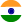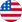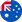24*7 support on WhatsAppChat NowProduct Edition:1st Edition
Author: David F. Anderson
Book Name: Introduction To Probability
Subject Name: Maths

# Introduction To Probability 1st Edition

0 out of 5.0
43 reviews

This classroom-tested textbook is an introduction to probability theory with the right balance between mathematical precision probabilistic intuition and concrete applications. Introduction to Probability covers the material precisely while avoiding excessive technical details. After introducing the basic vocabulary of randomness including events probabilities and random variables the text offers the reader a first glimpse of the major theorems of the subject: the law of large numbers and the central limit theorem. The important probability distributions are introduced organically as they arise from applications. The discrete and continuous sides of probability are treated together to emphasize their similarities. Intended for students with a calculus background the text teaches not only the nuts and bolts of probability theory and how to solve specific problems but also why the methods of solution work.Read more

5
-3
4
11
3
17
2
12
1
6

0

## Students who viewed this book also checked out